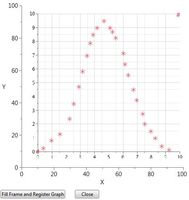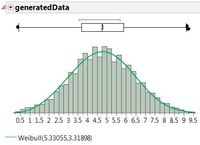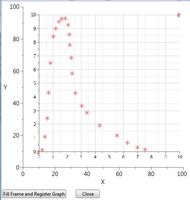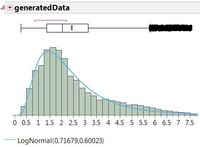Our World Statistics Day conversations have been a great reminder of how much statistics can inform our lives. Do you have an example of how statistics has made a difference in your life? Share your story with the Community!
Choose Language Hide Translation Bar

## Fit Probability Density Function From Sketch

This script is an extension of the "Click on an image to record (x,y) points" from the Data Table Tools Add-in by Brady Brady, JMP Division, SAS. It approximates the graph as a density so Distribution - Continous fit can convert it to an equation.

1. Choose a sketch of a probability function or blank grid.
2. Fill frame and set the x and y scales (e.g. 0,0 & 10,10).
3. Add points to approximate the desired probability function.
4. Press the close button...
5. (Fit Spline [Lambda 0.05] and save prediction formula).
6. (Exclude original data, add 200 rows incrementing X from its smallest to largest values)
7. (Normalise the generated predicted Y's and convert to a count such that the total count ~10,000)
8. (Create a new table with each increment of X duplicated the number of times indicated by step 6)
9. (Run Fit Continuous - All in the Distribution platform)
10. Choose the closest function with the correct properties and make a note of the hyperparameters.
11. Optional (JMP Pro): Use bootstrap to get an idea of the ranges the hyperparameters might take.Article Labels
Article Tags
Contributors